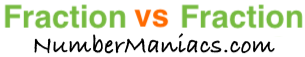3/4 vs 5/8People compare 3/4 vs 5/8 drywall, 3/4 vs 5/8 pipe, 3/4 vs 5/8 cup, 3/4 vs 5/8 ton, 3/4 vs 5/8 drill bit, 3/4 vs 5/8 poker, 3/4 vs 5/8 sump pump, 3/4 vs 5/8 garden hose, and many other items.

Is 3/4 greater than 5/8? Is 3/4 less than 5/8? What is the difference between 3/4 and 5/8? These and other questions are answered below so you can get a better perspective of 3/4 vs 5/8.

3/4 vs 5/8 Fractional Comparisons
3/4 is more than 5/8
The difference between 3/4 and 5/8 is 1/8
3/4 is 1/8 more than 5/8

3/4 vs 5/8 Decimal Comparisons
3/4 vs 5/8 as a decimal is 0.75 vs 0.625
The difference between 3/4 and 5/8 is 0.125
3/4 is 0.125 more than 5/8

3/4 vs 5/8 Percent Comparisons
3/4 vs 5/8 as a percent is 75% vs 62.5%
3/4 is 20 percent more than 5/8

Fraction vs Fraction
Submit another set of fractions that we can compare for you!

/
vs
/

The math behind the numbers
Calculations on this page are rounded to the nearest thousandth if necessary. Below is the math we used to analyze 3/4 vs 5/8:

To compare the size of 3/4 vs 5/8, we simply subtracted 5/8 from 3/4:

3/4 - 5/8 = |1/8|

To find 3/4 vs 5/8 as a decimal, we divided the numerator by the denominator for each fraction.

3 ÷ 4 = 0.75
5 ÷ 8 = 0.625

To find 3/4 vs 5/8 as a percent, we divided the numerator by the denominator and then multiplied the quotient by 100 for each fraction.

(3 ÷ 4) x 100 = 75%
(5 ÷ 8) x 100 = 62.5%

We found that 3/4 is 20 percent more than 5/8 by dividing the difference between the two fractions by the second fraction and then multiplied it by 100:

((1/8)/(5/8))×100 = 20%

That's it folks. We hope this page gave you a better understanding of 3/4 vs 5/8 with our explanations and conclusions.

3/4 vs 5/9
Here are the next two fractions we have compared and analyzed.